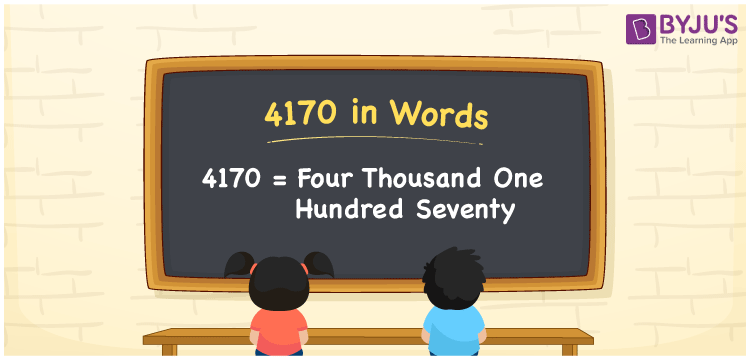# 4170 in Words

4170 in words is written as Four thousand one hundred seventy. In both the International System of Numerals and the Indian System of Numerals, 4170 is written as Four thousand one hundred seventy. The number 4170 is a Cardinal Number as it could represent some quantity. For example, “the area of this land is 4170 metres square”.

 4170 in Words Four thousand one hundred seventy Four thousand one hundred seventy in Number 4170

## 4170 in English Words

4170 in English words is read as “Four thousand one hundred seventy”.## How to Write 4170 in Words?

To write 4170 in words, we shall use the place value chart. In the place value chart, put 4 in the thousands, 1 in the hundreds, 7 in the tens, and 0 in the ones, respectively. Let us make a place value chart to write the number 4170 in words.

 Thousands Hundreds Tens Ones 4 1 7 0

Thus, we can write the expanded form as

4 × Thousand + 1 × Hundred + 7 × Ten + 0 × One

= 4 × 1000 + 1 × 100 + 7 × 10 + 0 × 1

= 4000 + 100 + 70 + 0

= 4170

= Four thousand one hundred seventy.

4170 is a natural number which is the successor of 4169 and the predecessor of 4171.

4170 in words – Four thousand one hundred seventy

• Is 4170 an odd number? – No
• Is 4170 an even number? – Yes
• Is 4170 a perfect square number? – No
• Is 4170 a perfect cube number? – No
• Is 4170 a prime number? – No
• Is 4170 a composite number? – Yes

## Frequently Asked Questions on 4170 in Words

Q1

### How to write 4170 in words?

4170 in words is written as Four thousand one hundred seventy.
Q2

### How to write 4170 in the International and Indian System of Numerals?

In both, the system of numerals, 4170 in words, is written as Four thousand one hundred seventy.
Q3

### How to write 4170 in a place value chart?

In the place value chart, write 4 in the thousands, 1 in the hundreds, 7 in the tens, and 0 in the ones, respectively.